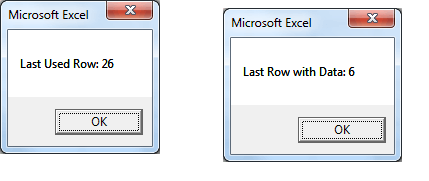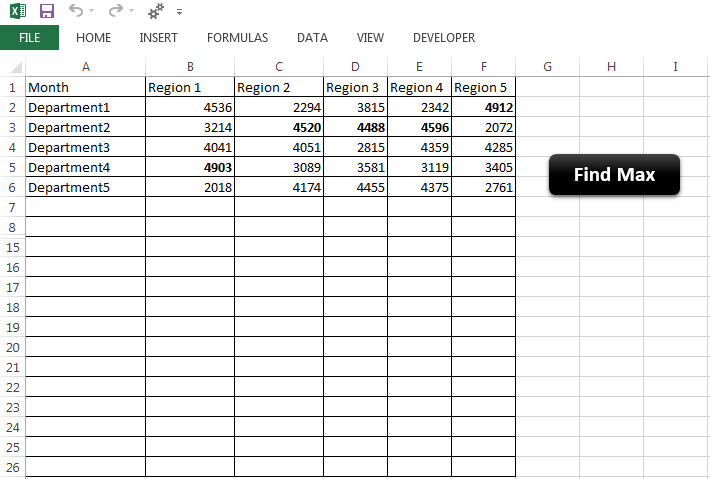### Description:

We need are required to find Last used Row with data if we want to perform certain task on each row of worksheet.If the number of rows are fixed or constant always suppose say 100, we can loop through the 100 rows and do what ever we want to apply. But if the rows are varying, we can loop through the rows to do our tasks by Finding Last Used Row with Data.

### Solution(s):

We can use .Cells.SpecialCells(xlLastCell).Row property to get last used Row. And we can loop through the Rows from back to check if any data exist.

##### Finding Last Used Row with Data – Example

The following example will show you how to find last row with data.

```Sub sblastRowOfASheet()
'In this example we are finding the last Row of an ActiveSheet

Dim lastRow As Long

lastRow = ActiveSheet.Cells.SpecialCells(xlLastCell).Row
MsgBox "Last Used Row: " & lastRow
lRow = ActiveSheet.Cells.SpecialCells(xlLastCell).Row
Do While Application.CountA(ActiveSheet.Rows(lRow)) = 0 And lRow <> 1
lRow = lRow - 1
Loop
lastRow = lRow
MsgBox "Last Row with Data: " & lastRow

End Sub
```
###### Instructions:
1. Open an excel workbook
2. Press Alt+F11 to open VBA Editor
3. Insert a New Module from Insert Menu
4. Copy the above code and Paste in the code window
5. To check, enter some data in a worksheet (suppose at D10) and delete and enter some data before this Row (suppose at G8). So, your last used row is 10 and Row with Data is 8.
6. Now Run a Macro, you should see a message box as shown below
###### Output##### Finding Last Used Row with Data – Case Study

The following example will show you real time example and when need find the last Row with data.

###### Requirement:
1. User wants to paste his data for 5 Regions
2. Number of Departments could be any thing between 1 to 25 (In this data it is 5 but it is not fixed- it will vary)
3. Find maxim sales from 5 Regions and Bold the Font
###### Code:
```
Sub sblastRowOfASheetFindMax()

Dim lastRow, lRow As Integer
Dim iCntr, jCntr, iMaxRow As Integer
Dim vMax

lastRow = ActiveSheet.Cells.SpecialCells(xlLastCell).Row
lRow = ActiveSheet.Cells.SpecialCells(xlLastCell).Row
Do While Application.CountA(ActiveSheet.Rows(lRow)) = 0 And lRow <> 1
lRow = lRow - 1
Loop
lastRow = lRow
Range(Cells(2, 2), Cells(lRow, 6)).Font.Bold = False

For iCntr = 1 To 5 'for fixed columns
vMax = 0
iMaxRow = 2
For jCntr = 2 To lRow ' for each row from 2nd row

If vMax < Cells(jCntr, iCntr + 1) Then
vMax = Cells(jCntr, iCntr + 1)
iMaxRow = jCntr
End If

Next

Cells(iMaxRow, iCntr + 1).Font.Bold = True
Next

End Sub

```
###### Explanation:

1. ActiveSheet.Cells.SpecialCells(xlLastCell).Row returns the last used Row of the active sheet

2. The below code finds the last used Row with data
```lRow = ActiveSheet.Cells.SpecialCells(xlLastCell).Row Do While Application.CountA(ActiveSheet.Rows(lRow)) = 0 And lRow <> 1 lRow = lRow - 1 Loop lastRow = lRow 'Last used row with data```

3. The first For loop is to loop through the fixed number of Columns (5), i.e Regions
```For iCntr = 1 To 5 'for fixed columns ... Next```
4. The second For loop is to loop through the rows (Rows are not fixed), the number of rows we found in step:2
```For jCntr = 2 To lRow ' for each row from 2nd row ... Next```

5. In second for loop we are finding the maximum value and changing the font to bold after this loop
```vMax = 0 iMaxRow = 2 For jCntr = 2 To lRow ' for each row from 2nd row```

``` ```

``` If vMax < Cells(jCntr, iCntr + 1) Then vMax = Cells(jCntr, iCntr + 1) iMaxRow = jCntr End If Next Cells(iMaxRow, iCntr + 1).Font.Bold = True```

###### Instructions:

Download the file below and lick on the FindMax button and Explore the Code.

###### Output

Here is the example screen shot.##### Finding Last Used Row with Data: Example File

Download the example file and see the example case of finding last used row with data.

ANALYSISTABS -Last Row with Data

120+ Professional Project Management Templates!

A Powerful & Multi-purpose Templates for project management. Now seamlessly manage your projects, tasks, meetings, presentations, teams, customers, stakeholders and time. This page describes all the amazing new features and options that come with our premium templates.

LIMITED TIME OFFER
ON SALE85% OFF

Excel Templates

120+ Project Management Templates Pack

Excel | PowerPoint | Word

ULTIMATE RESOURCE MANAGEMENT TEMPLATE

Excel Template

50+ Essential Project Management Templates

Excel | PowerPoint | Word

Project Portfolio Management Templates

Excel | PowerPoint Templates

50+ Excel Project Management Templates

Excel Templates

By Published On: February 8th, 2013Categories: Excel VBATags: